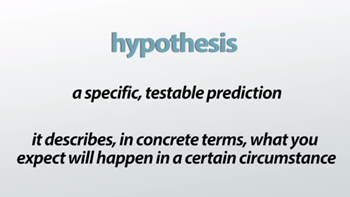# Describe briefly the logic underlying hypothesis testing

### October 29, 2019Describe briefly the logic underlying hypothesis testing
The logic of hypothesis testing is rooted in an understanding of the sampling distribution of the mean. ... We expect that, if the null hypothesis is true, then a random sample selected from a given population will have a sample mean equal to the value stated in the null hypothesis
What Is a Hypothesis?
Imagine you have a test at school tomorrow. You stay out late and see a movie with friends. You know that when you study the night before, you get good grades. What do you think will happen in tomorrow's test? When you answered this question, you formed a hypothesis. A hypothesis is a specific, testable prediction. It describes in concrete terms what you expect will happen in a certain circumstance. Your hypothesis may have been, 'If not studying lowers test performance and I do not study, then I will get a low grade on the test.'
The Purpose of a Hypothesis
A hypothesis is used in an experiment to define the relationship between two variables. The purpose of a hypothesis is to find the answer to a question. A formalized hypothesis will force us to think about what results we should look for in an experiment. The first variable is called the independent variable. This is the part of the experiment that can be changed and tested. The independent variable happens first and can be considered the cause of any changes in the outcome. The outcome is called the dependent variable. The independent variable in our previous example is not studying for a test. The dependent variable that you are using to measure outcome is your test score.

Let's use the previous example again to illustrate these ideas. The hypothesis is testable because you will receive a score on your test performance. It is measurable because you can compare test scores received from when you did study and test scores received from when you did not study.
A hypothesis should always:
1. Explain what you expect to happen
2. Be clear and understandable
3. Be testable
4. Be measurable
5. And contain an independent and dependent variable
6. How to Develop a Hypothesis
Another important aspect of a hypothesis is that it should be based on research. Remember that the purpose of a hypothesis is to find the answer to a question. The first thing you should do if you want to answer a question is to find as much information on the topic as you can. Before you come up with a specific hypothesis, spend some time doing research. Then, start thinking of questions you still have. After thoroughly researching your question, you should have an educated guess about how things work. This guess about the answer to your question is where your hypothesis comes from.

Let's imagine that you want to know why the leaves on the tree in your front yard change colour in the fall. First, you would research this phenomenon. You observe what you see happen and read about the subject. You discover that the colour change happens when the temperature cools. What question does this information make you ask? You come up with the following question: 'Does temperature cause the leaves to change colour on the tree in my front yard?' Next, you ask yourself if this can be tested. If it can be tested, you'll write a hypothesis that states what you expect to find. Your hypothesis could be 'If lower temperatures cause leaves to change colour and the temperature surrounding a tree is decreased, then the leaves will change colour.'
Introduction to Hypothesis Testing
Hypothesis Testing Hypothesis testing is a statistical procedure Allows researchers to use sample data to draw inferences about the population of interest Although the details of a hypothesis test will change from one situation to another, the general process will remain constant.

Hypothesis Testing (cont.) For this chapter, we have to understand z- scores, probability, and the distribution of sample means to create a new statistical procedure known as a hypothesis test.

Hypothesis Test A hypothesis test is a statistical method that uses sample data to evaluate a hypothesis about a population mean. Underlying logic State a hypothesis about a population State a hypothesis about a population Use the hypothesis to predict the characteristics that the sample should have to Use the hypothesis to predict the characteristics that the sample should have to Obtain a random sample from the population Compare the obtained sample data with the prediction that was made from the hypothesis Compare the obtained sample data with the prediction that was made from the hypothesis

A hypothesis test is typically used in the context of a research study Once a researcher completes a research study, a hypothesis test is used to evaluate the results Details of the hypothesis test will change from one situation to another detail of the hypothesis test will change from one situation to another For now, we will focus on the most common hypothesis tests

Situation: A researcher is using one sample to examine one unknown population The purpose of the research is to determine the effect of the treatment on the individuals in the population. The goal is to determine what happens to the population after the treatment is administered.FB Page

@ELITE_Mv